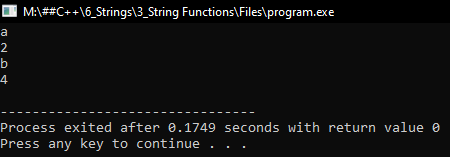# Built-in String Functions in C++

## Built-in String Functions in C++:

In this article, I am going to discuss Built-in String Functions in C++ Language with examples. Please read our previous article, where we discussed Reading and Writing Strings in C++ with examples. There is the header file called ‘cstring’ or ‘string.h’. These header files are having some built-in functions. Using those built-in functions, we can perform some operations on the string. So, let us discuss the built-in functions one by one with examples.

##### Strlen() String Function in C++: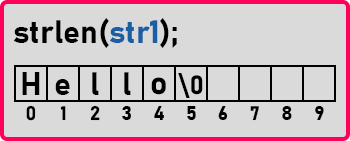Let’s say we have an array called ‘str1’. And we want to find out the length of this string. So, the length of this string is ‘5’ as there are 5 letters. And if you see the indices the indices are from 0 and the last index is 5. But at that index null character is present. So total 5 alphabets are there in this string. There is a function called ‘strlen()’. Using this strlen() function. we can find out the length of any string. Let us see the program for this one.

##### Program to find the string length using strlen function in C++:
```#include <iostream>
#include <cstring>
using namespace std;
int main()
{
char s;
cout << "Enter a String: ";
cin.getline (s, 30);
cout << "Length: " << strlen (s) << endl;
return 0;
}
```
###### Output: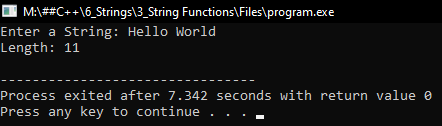##### Program to find the string length using loop in C++:
```#include<iostream>
using namespace std;
int main()
{
char str = "welcome";
int count = 0;
for (int i = 0; str[i] != '\0'; i++)
{
count++;
}
cout << "length is " << count << endl;
return 0;
}
```
###### Output: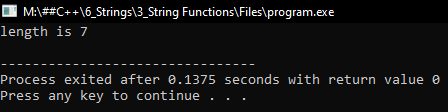##### Strcat (destination, source) String Function in C++:

The Next function is ‘stringcat’. This is for concatenating two strings in C++. We have to pass two strings as parameters that are two-character type arrays as parameters. The first parameter is ‘destination’ and the second parameter is ‘source’.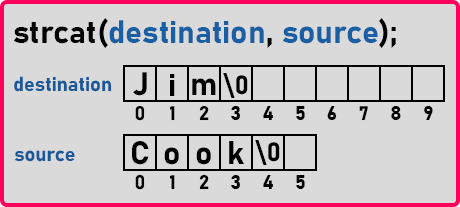So, there are two strings that are ‘Jim’ and ‘Cook’. We want to combine these two. We want to combine the source to the destination. After the end of the first string that is it is ending at ‘m’ then it should start writing ‘Cook’ here. And after the end, the null character should be included.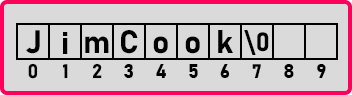So, this is what happens when we concatenate a string. Now it is a single large string and there is one more version of string concatenation that is ‘strncat’. ‘n’ is included here. In this function, we can mention how many characters we want to concatenate from the second string. The syntax for ‘strncat’ is strncat (destination, source, length)’.

Suppose we want only 3 letters of ‘Cook’ then we will right, strncat (destination, source, 3). Now let us see the program for it.

##### Program to concatenate two strings in C++:
```#include <iostream>
#include <cstring>
using namespace std;
int main ()
{
char s1 = "Jim";
char s2 = "Cook";
strcat (s1, s2);
cout << s1 << endl;
}
```
###### Output: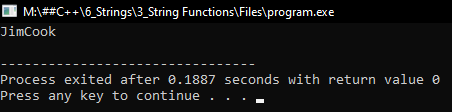##### Strcpt (destination, source) String Function in C++:

This function will copy the contents of one string to another string. So, there is a source and a destination. We want the contents of the source to be copied in the destination.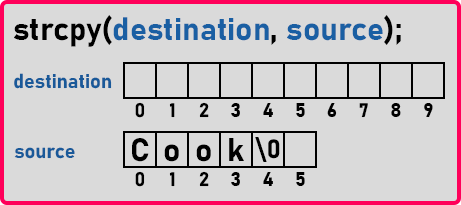Here we have a string ‘Cook’ in the source. We want to copy it into the destination. So, with the help of ‘strcpy’ this string will be copied in destination along with the null character. It needs two parameters, the first one is where we want to copy the string and the second is which string we want to copy. Let us see the program for this one.

##### Program to copy strings in C++:
```#include <iostream>
#include <cstring>
using namespace std;
int main()
{
char s1 = "";
char s2 = "Cook";
strcpy (s1, s2);
cout << s1 << endl;
}
```
###### Output: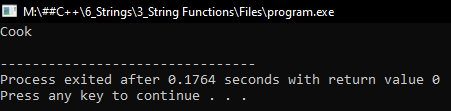##### Strstr (main, sub) String Function in C++:

This function is used for finding a substring from the main string. We can find out the existence of substring in the ‘main’ string. If ‘sub’ is found then it will give the occurrence of the substring followed by the remaining string.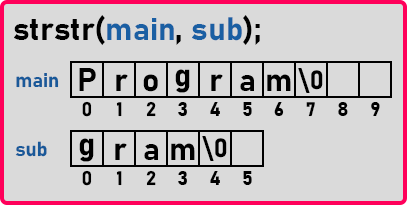So here “Program” is the main string. And below that, another string is there which is “gram”. So, we want to find out if there is any string called gram in the main string i.e. Program. Let us see this in the program.

##### Program to check for a substring in the main string in C++:
```#include <iostream>
#include <cstring>
using namespace std;
int main()
{
char s1 = "Programming";
char s2 = "gram";
cout << strstr (s1, s2) << endl;
}
```
###### Output: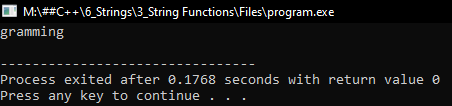If we give a word or letter which is not present in the main string then it will cause a runtime error. Because if the given word is not found in the main string, then ‘strstr’ will return null. So why there is an error? Because we are printing null in ‘cout’ statement. Let us write the program for it.

```#include <iostream>
#include <cstring>
using namespace std;
int main()
{
char s1 = "Programming";
char s2 = "l";

if (strstr (s1, s2) != NULL)
cout << strstr (s1, s2) << endl;
else
}
```
###### Output: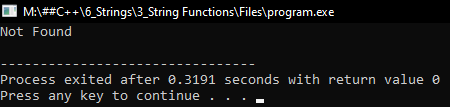##### Strchr (main, char) String Function in C++:

This function will find the occurrence of a given character in a string. It will take two parameters that is a string and the character for which we want the occurrence in the given string. Let us take an example.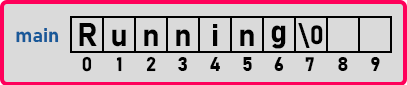If we write, ‘strchr (main, ‘n’)’ then this function will look for ‘n’ from the left-hand side in a string. It will take the occurrence of ‘n’ from the left-hand side. So, it will consider ‘n’ present at the index of ‘2’. Now if we want the right side ‘n’ then how we can get that? For that, there is another version of this function that is ‘strrchr’. There is a double ‘r’ in the function name. So, if we write ‘strrchr (main, ‘n’)’ then it will consider ‘n’ which is present at the index of ‘5’ that is from the right side. Let us see the program for this one.

##### Program to find the occurrence of a given character in a string in C++:
```#include <iostream>
#include <cstring>
using namespace std;
int main()
{
char s1 = "Running";
cout << strchr (s1, 'n') << endl;
}
```
###### Output: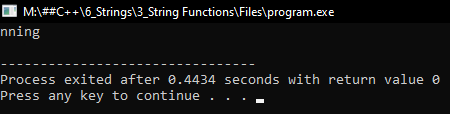##### Program for strrchr:
```#include <iostream>
#include <cstring>
using namespace std;
int main()
{
char s1 = "Running";
cout << strrchr (s1, 'n') << endl;
}
```
###### Output: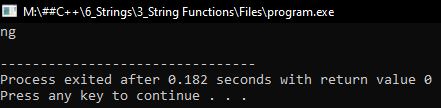##### Strcmp (str1, str2) String Function in C++: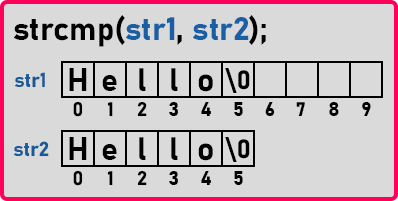Now, this function will compare strings in dictionary order or alphabetical order. So, the first one that appears in the dictionary is a smaller word and the second one is a larger word or a bigger word. Suppose there are two words, if we take two words that are ‘apple’ and ‘banana’. ‘apple’ is a smaller word than ‘banana’ because ‘banana’ comes after ‘apple.

If str1 comes first in the dictionary then it is smaller so it will return a negative value or it may be ‘-1’ also, it depends on the compiler. If the first string is equal to the second string it will return zero and if the first string is greater than the second string then it will return a positive value. Let us see this in the C/C++ program:

##### Program for string compare in C++:
```#include <iostream>
#include <cstring>
using namespace std;
int main()
{
char s1 = "Hello";
char s2 = "HEllo";
cout << strcmp (s1, s2) << endl;
}
```
###### Output: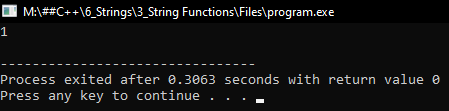##### Strtol (str1) and strtof (str1, NULL) Function in C++:

These functions are used for converting a string into a number.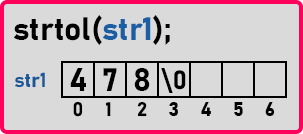String ‘str1’ is containing numbers. We can say this function as “string to long”. So, it will return a long integer out of this number. It will convert this string into a long integer.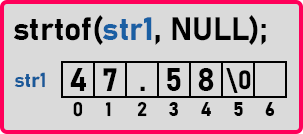And this is ‘strtof’ that is “string to float”. So, it will convert a string to a float number. So ‘str1’ has a float number here. So ‘strtof’ takes parameter a string and the second parameter is actually the end of a string so usually, it is taken as null. This is not a useful parameter but this type of this function is having one more parameter so just kept it null. Let us try these two functions in the program.

##### Program for Strtol (str1) and strtof (str1, NULL) Functions in C++:
```#include <iostream>
#include <cstdlib>
using namespace std;
int main()
{
char s1 = "478";
char s2 = "47.58";

long int a = strtol (s1, NULL, 10);
float b = strtof (s2, NULL);

cout << a << endl;
cout << b << endl;
}
```
###### Output: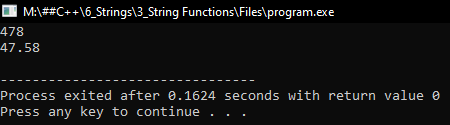##### Strtok (str1, “=;”) Function in C++:

Here the function is ‘strtok’ that is a string token. This will organize a string.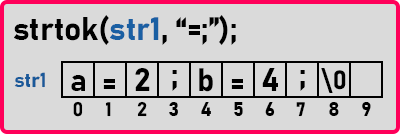If you observe, ‘str1’ contains two statements that are ‘a = 2;’ and ‘b = 4;’. These values are separated by semicolons. From this function, we want to know what is the value of ‘a’ or what is the value of ‘b’. We should be able to tokenize this one so what are the tokens? ‘a’ is one token and ‘2’ is another token and ‘b’ and ‘4’ are also tokens.

So, we want to tokenize this string, make the token out of it. For that, the delimiters are the symbols that are separating the ‘=’ symbol and the ‘;’ symbol. The string should be tokenized based on these two symbols that are ‘=’ and ‘;’.

The function ‘strtok’ will tokenize this string based on the symbols that we used in the parameter or delimiters that we use here. This type of string is famously known as key-value pair i.e. ‘a=2; b=4’. This is commonly used in programming. So let us look at a program for this one.

##### Program for Strtok (str1, “=;”) Function in C++:
```#include <iostream>
#include <cstdlib>
#include <cstring>
using namespace std;
int main()
{
char s1 = "a=2;b=4";
char *token = strtok (s1, "=;");
while (token != NULL)
{
cout << token << endl;
token = strtok (NULL, "=;");
}
}
```
###### Output: